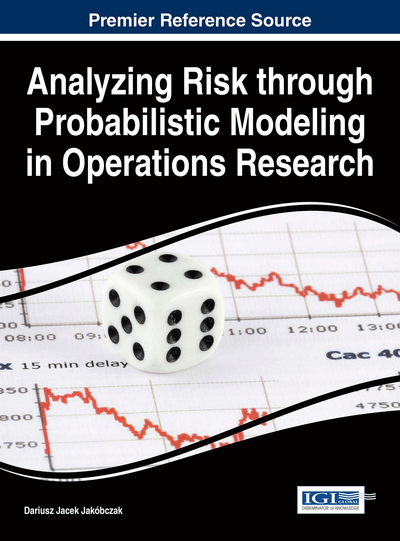# Data Extrapolation via Curve Modeling in Analyzing Risk: Value Anticipation for Decision Making

Dariusz Jacek Jakóbczak (Technical University of Koszalin, Poland)
DOI: 10.4018/978-1-4666-9458-3.ch001

## Abstract

Risk analysis needs suitable methods of data extrapolation and decision making. Proposed method of Hurwitz-Radon Matrices (MHR) can be used in extrapolation and interpolation of curves in the plane. For example quotations from the Stock Exchange, the market prices or rate of a currency form a curve. This chapter contains the way of data anticipation and extrapolation via MHR method and decision making: to buy or not, to sell or not. Proposed method is based on a family of Hurwitz-Radon (HR) matrices. The matrices are skew-symmetric and possess columns composed of orthogonal vectors. The operator of Hurwitz-Radon (OHR), built from these matrices, is described. Two-dimensional data are represented by the set of curve points. It is shown how to create the orthogonal and discrete OHR and how to use it in a process of data foreseeing and extrapolation. MHR method is interpolating and extrapolating the curve point by point without using any formula or function.
Chapter Preview
Top

## Background

The following question is important in mathematics and computer science: is it possible to find a method of curve interpolation and extrapolation in the plane without building the interpolation polynomials? This chapter aims at giving the positive answer to this question. Current methods of curve interpolation are based on classical polynomial interpolation: Newton, Lagrange or Hermite polynomials and spline curves which are piecewise polynomials (Dahlquist & Bjoerck, 1974). Classical methods are useless to interpolate the function that fails to be differentiable at one point, for example the absolute value function f(x) = |x|at x = 0. If point (0;0) is one of the interpolation nodes, then precise polynomial interpolation of the absolute value function is impossible. Also when the graph of interpolated function differs from the shape of polynomials considerably, for example f(x) = 1/x, interpolation is very hard because of existing local extrema of polynomial. We cannot forget about the Runge’s phenomenon: when interpolation nodes are equidistance then high-order polynomial oscillates toward the end of the interval, for example close to -1 and 1 with function f(x) = 1/(1+25x2) (Ralston, 1965). MHR method is free of these bad feature. Computational algorithm is considered and then it is important to talk about time. Complexity of calculations for one unknown point in Lagrange or Newton interpolation based on n nodes is connected with the computational cost of rank O(n2). Proposed method has lower calculation complexity.

A significant problem in risk analysis and decision making is that of appropriate data representation and extrapolation (Brachman & Levesque, 2004). Two-dimensional data can be treated as points on the curve. Classical polynomial interpolations and extrapolations (Lagrange, Newton, Hermite) are useless for data anticipation, because the stock quotations or the market prices represent discrete data and they do not preserve a shape of the polynomial. Also Richardson extrapolation has some weak sides concerning discrete data. This chapter is dealing with the method of data foreseeing and value extrapolation by using a family of Hurwitz-Radon matrices. The quotations, prices or rate of a currency, represented by curve points, consist of information which allows us to extrapolate the next data and then to make a decision (Fagin et al, 1995).

## Complete Chapter List

Search this Book:
Reset